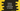# Python program to convert centimeter to inches## Python program to convert centimeter to inches:

In this post, we will learn how to convert a distance in centimeter to inches. This program will take one input from the user in centimeter and convert it to inches.

With this program, you will learn how to take user inputs, how to store user inputs, how to do small mathematical calculations, and how to print a value to the user.

### Algorithm:

1 inch is equal to 2.54 centimeters. So, if we divide the value that we are reading from the user by 2.54, it will give us the value in inches.

### Python program:

Below is the complete python program:

``````cm_distance = float(input("Enter the distance in cm: "))

inch_distance = cm_distance/2.54

print('Distance in inch: {}'.format(inch_distance))``````

Here,

• cm_distance is a variable used to store the distance in centimeter. It reads the value from the user and stores it in cm_distance.
• We are converting this value to inch by dividing cm_distance by 2.54.
• The last line is printing the distance in inches.

### Sample output:

If you run this program, it will print output as like below:

``````Enter the distance in cm: 10
Distance in inch: 3.937007874015748

Enter the distance in cm: 20
Distance in inch: 7.874015748031496``````

### Format upto two decimal places:

If you want to format it upto 2 decimal places, you can write your program as like below:

``````cm_distance = float(input("Enter the distance in cm: "))

inch_distance = cm_distance/2.54

print('Distance in inch: {0:.2f}'.format(inch_distance))``````

It will give output as like below:

``````Enter the distance in cm: 10
Distance in inch: 3.94

Enter the distance in cm: 20
Distance in inch: 7.87``````